Test: DFT Algorithm Computation - 1

# Test: DFT Algorithm Computation - 1

Test Description

## 10 Questions MCQ Test Signals and Systems | Test: DFT Algorithm Computation - 1

Test: DFT Algorithm Computation - 1 for Electrical Engineering (EE) 2023 is part of Signals and Systems preparation. The Test: DFT Algorithm Computation - 1 questions and answers have been prepared according to the Electrical Engineering (EE) exam syllabus.The Test: DFT Algorithm Computation - 1 MCQs are made for Electrical Engineering (EE) 2023 Exam. Find important definitions, questions, notes, meanings, examples, exercises, MCQs and online tests for Test: DFT Algorithm Computation - 1 below.
Solutions of Test: DFT Algorithm Computation - 1 questions in English are available as part of our Signals and Systems for Electrical Engineering (EE) & Test: DFT Algorithm Computation - 1 solutions in Hindi for Signals and Systems course. Download more important topics, notes, lectures and mock test series for Electrical Engineering (EE) Exam by signing up for free. Attempt Test: DFT Algorithm Computation - 1 | 10 questions in 10 minutes | Mock test for Electrical Engineering (EE) preparation | Free important questions MCQ to study Signals and Systems for Electrical Engineering (EE) Exam | Download free PDF with solutions
 1 Crore+ students have signed up on EduRev. Have you?
Test: DFT Algorithm Computation - 1 - Question 1

### Which of the following is true regarding the number of computations required to compute an N-point DFT?

Detailed Solution for Test: DFT Algorithm Computation - 1 - Question 1

Explanation: The formula for calculating N point DFT is given as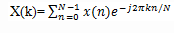From the formula given at every step of computing we are performing N complex multiplications and N-1 complex additions. So, in a total to perform N-point DFT we perform N2 complex multiplications and N(N-1) complex additions.

Test: DFT Algorithm Computation - 1 - Question 2

### Which of the following is true regarding the number of computations required to compute DFT at any one value of ‘k’?

Detailed Solution for Test: DFT Algorithm Computation - 1 - Question 2

Explanation: The formula for calculating N point DFT is given as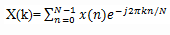From the formula given at every step of computing we are performing N complex multiplications and N-1 complex additions. So, it requires 4N real multiplications and 4N-2 real additions for any value of ‘k’ to compute DFT of the sequence.

Test: DFT Algorithm Computation - 1 - Question 3

### WNk+N/2=

Detailed Solution for Test: DFT Algorithm Computation - 1 - Question 3

Explanation: According to the symmetry property, we get WNk+N/2=-WNk.

Test: DFT Algorithm Computation - 1 - Question 4

The computation of XR(k) for a complex valued x(n) of N points requires:

Detailed Solution for Test: DFT Algorithm Computation - 1 - Question 4

Explanation: The expression for XR(k) is given as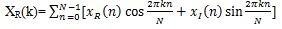So, from the equation we can tell that the computation of XR(k) requires 2N2 evaluations of trigonometric functions, 4N2 real multiplications and 4N(N-1) real additions.

Test: DFT Algorithm Computation - 1 - Question 5

Divide-and-conquer approach is based on the decomposition of an N-point DFT into successively smaller DFTs. This basic approach leads to FFT algorithms.

Detailed Solution for Test: DFT Algorithm Computation - 1 - Question 5

Explanation: T he development of computationally efficient algorithms for the DFT is made possible if we adopt a divide-and-conquer approach. This approach is based on the decomposition of an N-point DFT into successively smaller DFTs. This basic approach leads to a family of computationally efficient algorithms known collectively as FFT algorithms.

Test: DFT Algorithm Computation - 1 - Question 6

If the arrangement is of the form in which the first row consists of the first M elements of x(n), the second row consists of the next M elements of x(n), and so on, then which of the following mapping represents the above arrangement?

Detailed Solution for Test: DFT Algorithm Computation - 1 - Question 6

Explanation: If we consider the mapping n=Ml+m, then it leads to an arrangement in which the first row consists of the first M elements of x(n), the second row consists of the next M elements of x(n), and so on.

Test: DFT Algorithm Computation - 1 - Question 7

If N=LM, then what is the value of WNmqL

Detailed Solution for Test: DFT Algorithm Computation - 1 - Question 7

Explanation: We know that if N=LM, then WNmqL= WN/Lmq= WMmq.

Test: DFT Algorithm Computation - 1 - Question 8

How many complex multiplications are performed in computing the N-point DFT of a sequence using divide-and-conquer method if N=LM?

Detailed Solution for Test: DFT Algorithm Computation - 1 - Question 8

Explanation: The expression for N point DFT is given as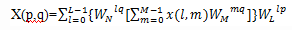The first step involves L DFTs, each of M points. Hence this step requires LM2 complex multiplications, second require LM and finally third requires ML2. So, Total complex multiplications= N(L+M+1)

Test: DFT Algorithm Computation - 1 - Question 9

How many complex additions are performed in computing the N-point DFT of a sequence using divide-and-conquer method if N=LM?

Detailed Solution for Test: DFT Algorithm Computation - 1 - Question 9

Explanation: The expression for N point DFT is given as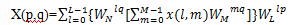The first step involves L DFTs, each of M points. Hence this step requires LM(M-1) complex additions, second step do not require any additions and finally third step requires ML(L-1) complex additions. So, Total number of complex additions= N(L+M-2).

Test: DFT Algorithm Computation - 1 - Question 10

Which is the correct order of the following steps to be done in one of the algorithm of divide and conquer method?
1) Store the signal column wise
2) Compute the M-point DFT of each row
3) Multiply the resulting array by the phase factors WNlq.
4) Compute the L-point DFT of each column.
5) Read the result array row wise.

Detailed Solution for Test: DFT Algorithm Computation - 1 - Question 10

Explanation: According to one of the algorithm describing the divide and conquer method, if we store the signal in column wise, then compute the M-point DFT of each row and multiply the resulting array by the phase factors WNlq and then compute the L-point DFT of each column and read the result row wise.

## Signals and Systems

32 videos|76 docs|63 tests
 Use Code STAYHOME200 and get INR 200 additional OFF Use Coupon Code
Information about Test: DFT Algorithm Computation - 1 Page
In this test you can find the Exam questions for Test: DFT Algorithm Computation - 1 solved & explained in the simplest way possible. Besides giving Questions and answers for Test: DFT Algorithm Computation - 1, EduRev gives you an ample number of Online tests for practice

## Signals and Systems

32 videos|76 docs|63 tests